## TN State Board 11th Chemistry Important Questions Chapter 5 Alkali and Alkaline Earth Metals

Question 1.
Explain why atomic and ionic radii increases from lithium to caesium?
On moving down the group, there is an increase in the number of shells, as well as increase in nuclear charge. The force of attraction between the nucleus and the added electron decreases. Hence, ionic and atomic radii increases.

Question 2.
Why alkali metals do not occur free in native?
Since ionisation energy values of alkali metals are low, they are very reactive. They readily combine with oxygen, moisture and CO2 of the atmosphere and form compounds. Hence they do not occur free in nature.Question 3.
Explain the following:
(i) Alkali metals have the lowest ionisation enthalpy compared to other elements in the respective period.
(ii) The second ionisation enthalpy values of alkali metals are high.
(i) The atoms of alkali metals have largest size in their respective periods and therefore valence electrons are loosely bond to the nucleus and hence Can be easily
(ii) The removal of an electron from the alkali metals gives’ monovalent cations having stable electronic configurations similar to the noble gas. Therefore, it becomes very difficult to remove the second electron from the stable configurations already attained. Hence, the second ionisation enthalpy values of alkali metals are high.

Question 4.
What do you understand by the term “hydration enthalpy”? Explain how the hydration enthalpy of alkali metal cations vary in the group.
The metal ion, in an aqueous solution gets surrounded by water dipoles in such a way that the cation or an anion is attracted by the opposite end of water dipoles. This process is known as hydration.

M+ (aq) + aq → [M (aq)]+ + energy

This process releases energy. This energy is known as hydration energy or hydration enthalpy.

Smaller the cation, greater is the degree of hydration. Thus, the degree of hydration of M+ ions decreases from Li+ ion to Cs+ ions.Question 5.
Lithium salts are more soluble than the other metals of group 1. Explain why?
The high solubility of Li salts is due to strong solvation of small size of Li+ ion.

Question 6.
Alkali metals give a characteristics colour for a bunsen flame. Explain why?
The heat in the flame excites the valence electron to a higher energy level. When it drops back to its actual energy level, the excess energy is emitted as light, whose wavelength is in the visible region. This appears as a characteristic colour to the particular metal.

Question 7.
Bring out the similarities between lithium and magnesium.
(i) Both lithium and magnesium are harder than other elements in the respective groups. .
(ii) Lithium and magnesium react slowly with water. Their oxides and hydroxides are much less soluble and their hydroxides decompose on heating.
(iii) Both form a nitride, Li3N and Mg3N2, by direct combination with nitrogen. .
(iv) They do not give any superoxides and form only oxides, Li2O and MgO.
(v) The carbonates of lithium and magnesium decompose upon heating to form their respective oxides and CO2.
(vi) Lithium and magnesium do not form bicarbonates.
(vii) Both LiCl and MgCl2 are soluble in ethanol and are deliquescent. They crystalline form aqueous solution as hydrates, LiCl . 2H2O and MgCl2 . 8 H2OQuestion 8.
What is the cause for the distinctive behaviour of lithium? Compare the properties of lithium with other elements of the group.
The distinctive behaviour of Li+ ion is due to its exceptionally small size, high polarising power, high hydration energy and non availability of d-orbitals.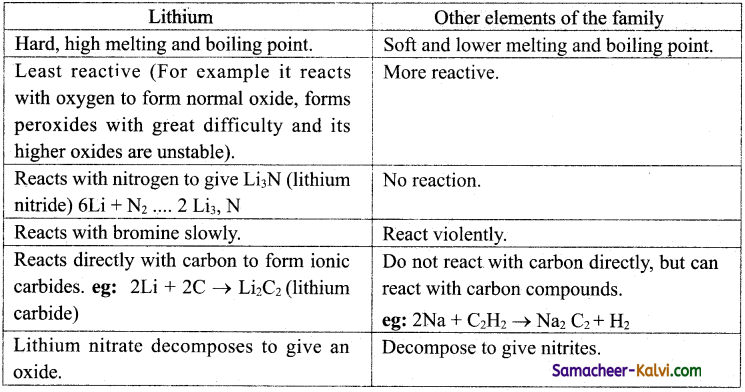Question 9.
Explain why alkali metals are very reactive.
The reactivity of alkali metals is due to their low ionisation enthalpy. The ionisation enthalpy decreases down the group. Hence the reactivity of alkali metal increases from Li to Cs.

The high reactivity of alkali metals is proved by their reaction with oxygen and halogen which are most electronegative.

Question 10.
Explain the action of alkali metals with oxygen.
All the alkali metals on exposure to air or oxygen bum vigorously, forming oxides on their surface. Lithium forms only monoxide, sodium forms the monoxide and peroxide and the other elements form monoxide, peroxide and superoxides. These oxides are basic in nature.

4 Li + O2 → Li2O (simple oxide)
2 Na + O2 → Na2O2 (peroxide)
M + O2 → MO2
(M = K, Rb,Cs; MO2 – superoxide)Question 11.
Explain why?
(i) The ionic character of hydrides of alkali metals increase from Li to Cs.
(ii) The stability of hydrides decrease down the group.
(iii) Hydrides behave as strong reducing agents.
(i) The electropositive character increases from Li to Cs. Hence their ionic character increases down the group.
(ii) The stability of the hydrides decreases down the group, as the size of the metal ion increases. The M—H bond distance increases with increase in size of metal
ion and it can be readily broken, i.e., the metal hydride is least stable.
(iii) They are powerful reducing agents as M—H bond is weaker and it can be readily broken.

Question 12.
Lithium iodide is covalent. Explain why?
Lithium iodide shows covalent character, as Li+ ion, being smaller exerts high polarising power on the iodide anion. Alternatively, the iodide ion being the largest can be polarised to a greater extent by Li+ ion. Hence, lithium iodide is covalent.Question 13.
Alkali metals dissolve in liquid ammonia and given deep blue solution. Explain the magnetic behaviour of these solution?
The alkali metal atom readily loses its valence electron in ammonia solution. Both the cation and the electron are ammoniated to give ammoniated cation and ammoniated electron.

M + (x + y)NH3 → [M(NH3)x]+ + [e(NH3)y]

The blue colour of the solution is due to the ammoniated electron which absorbs energy in the visible region of light and thus imparts blue colour to the solution. The solutions are paramagnetic and on standing slowly liberate hydrogen resulting in the formation of an amide.
M+ + e + NH3 → MNH2 + V2H2

In concentrated solution, the blue colour changes to bronze colour and become diamagnetic.Question 14.
Complete and balance the following equations.
(i) Li + H2O →
(ii) Na + C2H5OH →
(iii) CH ≡ CH + Na →
(i) 2Li + 2H2O → 2LiOH + H2 (Lithium hydroxide)
(ii) 2Na + 2C2H5OH → C2H5ONa + H2 (sodium ethoxide)
(iii) CH ≡ CH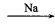HC ≡ CNaNaC ≡ C – Na (sodium acetylide)

Question 15.
Mention the uses of lithium.
(i) Lithium metal is used to make alloys.
(ii) Lithium is also used to make electro chemical cells.
(iii) Lithium carbonate is used in medicines.

Question 16.
Mention the uses of sodium.
(i) Sodium is used to make Na/Pb alloy needed to make Pb(Et)4 and Pb(Me)4.
(ii) Liquid sodium metal is used as a coolant in fast breeder nuclear reactors.

Question 17.
What are normal oxides, peroxides and superoxides? Give examples.
Normal oxides are those which contain O-2 ion.
eg: Na2O, BaO, Al2O3 etc.,
Peroxides contain —O—O— linkage. (O2 ions). The produce hydrogen peroxide on treatment with dilute acid.
Na2O2 + H2SO4 → Na2SO4 + H2O2
Superoxides contain greater proportion of oxygen than normal oxides and peroxides. They contain 02~ ion.
eg: KO2, RbO2 or CsO2.Question 18.
Give equation for the reaction of the following with water.
(i) Na2O,
(ii) K2O2,
(iii) KO2.
(i) Na2O + H2O → 2NaOH + H2O
(ii) K202 + 2H2O → 2KOH + H2O2
(iii) 2K02 + 2H2O → 2KOH + H2O2 + O2

Question 19.
Give equation for the preparation of sodium chloride from
(i) NaOH,
(ii) Na2O,
(iii) Na2CO3.
(i) NaOH + HCl → NaCl + H2O
(ii) Na2O + 2HCl → 2NaCl + H2O
(iii) Na2CO3 + 2HCl → 2NaCl + H2O + CO2

Question 20.
Which is more readily formed CSCl or NaCl, by the reaction of metal with chlorine? Explain.
Caesium chloride is more readily, formed than sodium chloride, because caesium is more electropositive than sodium. As the electropositive character of the alkali metal increases, the case of formation of the halide increases.Question 21.
Account for the low solubility of lithium luoride in water.
The low solubility of LiF in water is due to its high lattice enthalpy (small size of Li+ and F ) than its solvation (hydration) energy.

Question 22.
Lithium halides are covalent. Explain.
Due to the smaller size of lithium ion, it can polarise the larger halide ion to a greater extent. Hence Lithium halides are covalent.

Question 23.
Account for the thermal stability of carbonates and bicarbonates of alkali metals.
As the electropositive character increases down the group, the stability of the carbonates and bicarbonates increases. This is due to the decrease in polarising power of alkali metal cations. The carbonates (M2CO3) of alkali metals are remarkably stable up to 1273 K, above which they first melt and then eventually decompose to form oxides.Question 24.
Explain why lithium carbonate is the least stable among alkali metal carbonates.
Li2CO3 is considerably less stable and decomposes readily.
Li2CO3 Li2O + CO2 This is presumably due to large size difference between Li+ and CO23 which makes the crystal lattice unstable.

Question 25.
Account for the solubility of alkali metal carbonates and bicarbonates in water.
All the carbonates and bicarbonates are soluble in water and their solubilities increase rapidly on descending the group. This is due to the reason that lattice energies decrease more rapidly than their hydration energies on moving down the group.

Question 26.
Explain the solvay process of manufacturing sodium carbonate.
In this process, ammonia is converted into – ammonium carbonate which then converted to ammonium bicarbonate by passing excess carbon dioxide in a sodium chloride solution saturated with ammonia. The ammonium bicarbonate thus formed reacts with the, sodium chloride to give sodium bicarbonate and ammonium chloride. As sodium bicarbonate has poor solubility, it gets precipitated. The sodium bicarbonate is isolated and is heated to give sodium carbonate. The equations involved in this process are,

2NH3 + H2O + CO2 → (NH4)2CO3
(NH4)2CO3 + H2O + CO2 → 2NH4HCO3
NH4HCO3 + NaCl → NH4Cl + NaHCO3
2 NaHCO3 → Na2CO3 + CO4 + H4O

The ammonia used in this process can be recovered by treating the resultant ammonium chloride solution with calcium hydroxide. Calcium chloride is formed as a by-product.Question 27.
Mention the uses of sodium carbonate.
(i) Sodium carbonate known as washing soda is used heavily for laundering.
(ii) It is an important laboratory reagent used in the qualitative analysis and in volumetric analysis.
(iii) It is also used in water treatment to convert the hard water to soft water.
(iv) It is used in the manufacturing of glass, paper, paint etc…

Question 28.
How is pure sodium chloride prepared from crude salt?
Pure sodium chloride can be obtained from crude salt as follows. The insoluble impurities are removed by filtration from the crude salt solution with minimum amount of water. The filtrate contains sodium chloride solution. From this, sodium chloride can be crystallised by passing HCl gas into this solution. Calcium and magnesium chloride, being more soluble than sodium chloride, remain in solution.

Question 29.
Mention the uses of sodium chloride.
(i) It is used as a common salt or table salt for domestic purpose.
(ii) It is used for the preparation of many inorganic compounds such as NaOH and Na2CO3.Question 30.
Explain the Castner-Kellner process of manufacturing sodium hydroxide.
Sodium hydroxide is prepared commercially by the electrolysis of brine solution in Castner-Kellner cell using a mercury cathode and a carbon anode. Sodium metal is discharged at the cathode and combines with mercury to form sodium amalgam. Chlorine gas is evolved at the anode. The sodium amalgam thus obtained is treated with water to give sodium hydroxide.
At cathode: Na+ + e → Na(amalgam)
At anode: Cl → 1/2 Cl2↑ + e
2Na(amalgam) + 2H2O → 2NaOH + 2Hg + H2

Question 31.
Mention the uses of sodium hydroxide.
(i) Sodium hydroxide is used as a laboratory reagent.
(ii) It is also used in the purification of bauxite and petroleum refining.
(iii) It is used in the textile industries for mercerising cotton fabrics.
(iv) It is used in the manufacture of soap, paper, artificial silk and a number of chemicals.

Question 32.
How is sodium bicarbonate prepared?
This compound is prepared by saturating a solution of sodium carbonate with carbon dioxide. The white crystalline powder of sodium bicarbonate, being less soluble, precipitated out.Question 33.
Mention the uses of sodium bicarbonate.
(i) Primarily used as an ingredient in baking.
(ii) Sodium hydrogen carbonate is a mild antiseptic for skin infections.
(iii) It is also used in fire extinguishers.

Question 34.
Discuss the biological importance of sodium and potassium.
(i) Sodium and potassium ions maintain the ion balance and nerve impulse conduction.
(ii) Transport of sugar and amino acids into cells.
(iii) Potassium ions activate many enzymes, participate in the oxidation of glucose to produce ATP.
(iv) With sodium, potassium ion is responsible for the transmission of nerve signals.
(v) Sodium and potassium pump play an important role in transmitting nerve signals.

Question 35.
Why group 2 elements are called alkaline earth metals?
These elements with the exception of beryllium are commonly known as the alkaline earth metals because their oxides and hydroxides are alkaline in nature and these metal oxides are found in the earth’s crust.

Question 36.
Write the electronic configuration of group 2 elements.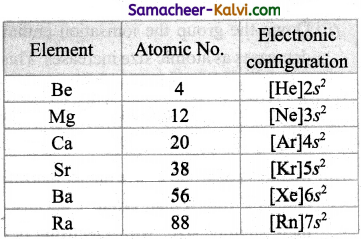Question 37.
Account for
(i) The atomic and ionic radii of group 2 elements are smaller than alkali metals.
(ii) The atomic and ionic radii increases down the group.
(i) The atomic and ionic radii of alkaline earth metals are smaller than the corresponding members of the alkali metals. This is due to the fact the Group 2 elements having a higher nuclear charge that allows electrons to be attracted more strongly towards the nucleus.
(ii) On moving down the group, the radii increases due to gradual increase in the number of the shells and the screening effect.

Question 38.
Explain why alkaline earth metals exhibit an oxidation state of +2.
The group 2 elements have two electrons in their valence shell and by losing these electrons, they acquire the stable noble gas configuration. So these elements exhibit +2 oxidation state in their compounds.

Question 39.
Explain:
(i) ionisation enthalpy of alkaline earth metals are low compared to ‘p’ block elements.
(ii) Ionisation enthalpy decreases down the group.
(iii) Ionisation enthalpy of group 2 elements are higher than that of group 1 elements.
(i) Due to a fairly large size of the atoms, alkaline earth metals have low ionisation enthalpies when compared to ‘p’ block elements.
(ii) Down the group the ionisation enthalpy decreases as atomic size increases. This is due to the addition of new shells as well as increase in the magnitude of the screening effect of inner shell electrons.
(iii) Members of group 2 have higher ionization enthalpy values than group 1 because of their smaller size, with electrons being more attracted towards the nucleus of | the atoms. Correspondingly they are less electropositive than alkali metals.Question 40.
Although IE1 values of alkaline earth metals ire higher than that of alkali metals the IE2 values of alkaline earth metals are much smaller than alkali metals. Explain.
This is because in alkali metals the second electron is to be removed from a cation, which has already acquired a noble gas configuration. In the case of alkaline earth metals, the second electron is to be removed from a monovalent cation, which still has one electron in the outermost shell. Thus, the second electron can be removed more easily in the case of group 2 elements than in group 1 elements.

Question 41.
Hydration enthalpies of ine earth metal ions are larger than these of alkali metal ions. Explain.
Smaller the size of the ions, greater is the extent of hydration. Hence alkaline earth metal ions are smaller in size compared to alkali metals. Hence their hydration enthalpies are much larger than alkali metals.

Question 42.
Account for the decrease in hydration enthalpy of group 2 elements down the group.
The hydration enthalpies of alkaline earth metal ions also decrease with increase in ionic size down the group.
Be > Mg > Ca > Sr > Ba
eg: MgCl2 and CaCl2 exist as MgCl2.6H2O and CaCl2 . 6H2O while NaCl and KCl do not form such hydrates.Question 43.
Account for the characteristic colour produced by the alkaline earth metal salt:: in bunsen flame.
The heat in the flame excites the valence electron to a higher energy level, when it drops back to its actual energy level, the excess energy is emitted as light, whose wavelength is in the visible region as shown in the table.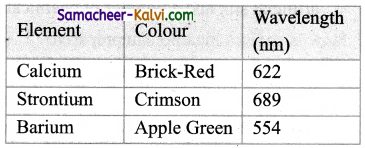Question 44.
Discuss the anomalous behaviour of beryllium.
The anomalous properties of beryllium is mainly due to its small size, high electronegativity, high ionisation energy
and high polarising power compared to the other elements in the group. The anomalous properties of beryllium compared to other elements of the group are mentioned in table.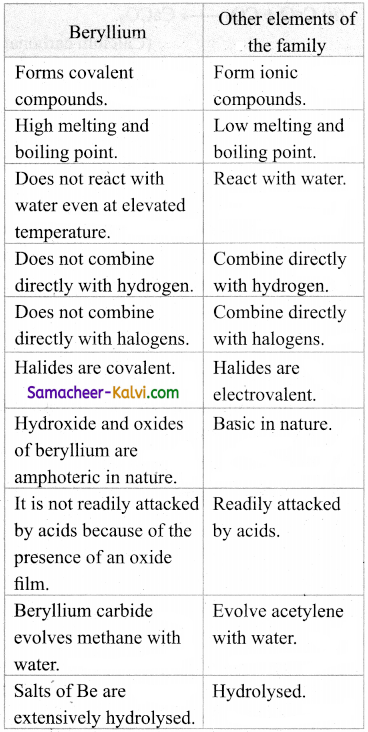Question 45.
What is the cause for diagonal relationship between beryllium and aluminium?
Beryllium (the first member of group (2) shows a diagonal, relatiofiship with aluminium. In this case, the size of these ions (rBe2+ = 0.45 Å and rAl3+= 0.54 Å) is not as close. However, their charge per unit area is closer (Be2+ = 2.36 and Al3+ = 2.50). They also have sameelectronegativity values (Be = 1.5; Al = 1.5).

Question 46.
Give methods of preparation of beryllium chloride.
Thermal decomposition of (NH4)2BeF4 is the best route for the preparation of BeF2. BeCl2 is conveniently made from the oxide.
BeO + C + Cl2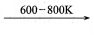BeCl2 + CO

Question 47.
How is beryllium hydride prepared? Give equation.
BeH2 can be prepared by the reaction of BeCl2 with LiAlH4 (Lithium aluminium hydride).
2BeCl2 + LiAlH4 » 2BeH2 + LiCl + AlCl3Question 48.
How does the basic nature of the oxides of group 2 elements vary down the group?
The basic nature of oxides increases down the group.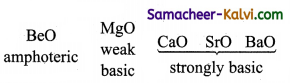Question 49.
How does (i) solubility,
(ii) thermal stability,
(iii) basic character hydroxides of group 2 elements vary down the group.
All the properties increases down the group.
Be(OH)2 < Mg(OH)2 < Ca (OH)2 < Sr (OH)2 < Ba (OH)2

Question 50.
Beryllium halide are covalent. Explain why?
Due to the smaller size of Be+2 ion, it polarises the halide ion to a greater extent. Hence Beryllium halides are covalent.Question 51.
Explain with equations beryllium hydroxide is amphoteric.
Beryllium hydroxide is amphoteric in nature as it reacts with both acid and alkali.
Be(OH)2 + 2 NaOH → Na2BeO2 +2H2O
Be(OH)2 + 2HCl → BeCl2 + 2H2O

Question 52.
Give a brief account of the structure of beryllium chloride.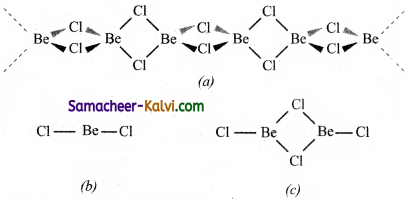(i) Beryllium chloride has a chain structure in the solid state as shown in structure-(a).
(ii) In the vapour phase BeCl2 tends to form a chloro-bridged dimer in structure-(c).
(iii) Which dissociates into the linear monomer at high temperatures of the order of 1200 K. structure-(b).

Question 53.
How does (i) the solubility and
(ii) the thermal stability of the carbonates of group 2 elements vary down the group.
(i) The solubility of carbonates in water decreases down the group.
(ii) The thermal stability increases down the group with increasing cationic size.

Question 54.
Explain why BeSO4 and MgSO4 are readily soluble in water.
The greater hydration enthalpies of Be2+ and Mg2+ ions overcome the lattice enthalpy factor and therefore their sulphates are soluble in water.Question 55.
Arrange the following as indicated.
(i) BeSO4, MgSO4 CaSO4, SrSO4 and BaSO4 in the increasing order of solubility,
(ii) Be(NO3)2, Mg(NO3)2, Ca(NO3)2, Sr (NO3)2, Ba(NO3)2 < Ba (NO3)2 in decreasing order of forming hydrated salts.
(i) BeSO4 > MgSO4 > CaSO4 > SrSO4 > BaSO4.
(ii) Be(NO3)2 < Mg(NO3)2 < Ca (NO3)2 < Sr(NO3)2 < Ba (NO3)2 [due to increase in size of M2+ ions].

Question 56.
How is quick lime produced on a commercial scale?
It is produced on a commercial scale by heating limestone in a lime kiln in the temperature range 1070-1270K.
CaCO3 → CaO + CO2
The reactionbeing reversible, carbon dioxide is removed as soon as it is produced to enable the reaction to proceed to completion.Question 57.
Give equations for the reaction of CaO with
(i) H2O, (ii) CO2, (iii) SiO2, (iv) P4O10.
(i) CaO + H2O → Ca(OH)2 (Calcium hydroxide)
(ii) CaO + CO2 → CaCO3 (Calcium carbonate)
(iii) CaO+ SiO2 → CaSiO3 (Calcium silicate)
(iv) 6 CaO + P4O10 → 2 Ca3(PO4)2 (Calcium phosphate)

Question 58.
What is slaked lime? How is it produced?
Calcium hydroxide (Ca(OH)2) is called slaked lime. It is formed by the addition of limited amount of water as quick lime.
CaO + H2O → Ca(OH)2

Question 59.
Mention the uses of calcium oxide (CaO).
(i) To manufacture cement, mortar and glass.
(ii) In die manufacture of sodium carbonate and slaked lime.
(iii) In the purification of sugar.
(iv) As a drying agent.Question 60.
(i) What happens when carbon dioxide is passed through lime water? Give equation.
(ii)What happens when excess of carbondioxide is passed through lime water? Give equation.
(i) When carbon dioxide is passed through lime water, it turns milky due to the formation of calcium carbonate.
Ca(OH)2 + CO2 → CaCO3↓ + H2O
(ii) On passing excess of carbon dioxide, the precipitate dissolves to form calcium hydrogen carbonate.
CaCO3 + CO2 + H2O → Ca(HCO3)2

Question 61.
Mention the uses of calcium hydroxide.
Calcium hydroxide is used:
(i) In the preparation of mortar, a building material.
(ii) In white washing due to its disinfectant nature.
(iii) In glass making, in tanning industry, in the preparation of bleaching powder and for the purification of sugar.Question 62.
Gypsum becomes less soluble in water as the temperature increases. This is known as retrograde solubility, which is a distinguishing characteristic of gypsum.

Question 63.
Explain the term ‘desert rose’.
Gypsum crystals are sometimes found to occur in a form that resembles the petals of a flower. This type of formation is referred to as ‘desert rose’, as they mostly occur in arid areas or desert terrains.

Question 64.
Explain why gypsum is used in making dry walls or wall board.
Gypsum is known to have low thermal conductivity, which is the reason why it is used in making drywalls dr wallboards. Gypsum is also known as a natural insulator.

Question 65.
How is plaster of paris’ produced from gypsum? Mention its use.
Gypsum is heated to about 300 degree Fahrenheit to produce plaster of Paris, which is also known as gypsum plaster. It is mainly used as a sculpting material.Question 66.
Explain the use of gypsum in agriculture.
It helps loosen up compact or clay soil and provides calcium and sulphur, which are essential for the healthy growth of a plant. It can also be used for removing sodium from soils having excess salinity.

Question 67.
What is ‘dead burnt plaster’? What is use?
Anhydrous calcium sulphate formed by heating plaster of Paris above 393 K is known a dead burnt plaster. It has a remarkable property of setting with water. On mixing adequate quantity of water, it forms a plastic mass that gets into a hard solid in 5 to 15 minutes.

Question 68.
Briefly outline the biological importance of calcium and magnesium.
(i) Mg is a cofactor of all enzymes that utilise ATP in phosphate transfer and energy release.
(ii) Magnesium is essential for DNA synthesis and is responsible for the stability and proper functioning of DNA.
(iii) Deficiency of magnesium results into, convulsion and neuro muscular irritation.
(iv) Calcium is present in blood and its concentration is maintained by hormones calcitonin and parathyroid hormone.
(v) Deficiency of calcium is blood causes it take to much time to clot and in muscle contraction.Question 69.
Explain what to meant by efflorescence.
It is a phenomenon by which water of hydration of a hydrated salt is lost on exposure to atmosphere, eg: Washing soda (Na2CO3 10H2O), and Glauber salt (Na2SO4 10H2O) when exposed to atmosphere lost their water of hydration and becomes powdery.

Question 1.
Among alkali metals, the metals having smallest metallic radius and largest metallic radius are:
(a) Li, Na
(b) Cs, Li
(c) Li, Cs
(d) Na, K
(c) Li, Cs
Hint:
On moving down the group, the metallic radius increases.

Question 2.
The melting points of alkali metals:
(a) increase down the group
(b) decrease down the group
(c) does not show a regular trend
(d) increases upto K and then decreases
(b) decrease down the group
Hint:
The low melting point is attributed to low binding energies of their .atoms in the crystal lattice an account of single electron in the valency shell and large atomic sizes. The binding energy decrease from Li to Cs consequently the melting points.
[ Note: The same reason is applicable to boiling points also.]Question 3.
Choose the correct statement:
(a) Alkali metals are highly electropositive because of their low ionisation energies.
(b) Alkali metal cations have stable electronic configuration.
(c) The hydration enthalpies of alkali metals decreases from Li+ to Cs+.
(d) all the above.
(d) all the above

Question 4.
Sodium react with water more vigorously than lithium because:
(a) it has higher atomic mass
(b) it is more electropositive
(c) it is more electronegative
(d) it is a metal
(b) it is more electropositive
Hint:
The reactivity of alkali metals towards water increases from Li to Cs. This is due to increase in electropositive character in the same order.Question 5.
Select the correct statement:
(a) Lithium carbonate is soluble in water.
(b) Potassium carbonate is soluble in water.
(c) Barium carbonate soluble in water.
(d) Bicarbonate of lithium is soluble in water.
(b) Potassium carbonate is soluble in water.
Hint:
(a) The lattice energy of Li2CO3 is high compared to the solvation energy. Hence it is insoluble in water.
(b) Correct statement.
(c) BaCO3 is insoluble in water due to the high lattice energy.
(d) Lithium, due to its less electropositive nature does not form a bicarbonate.

Question 6.
Which of the following has the lowest thermal stability?
(a) Li2CO3
(b) Na2CO3
(c) K2CCO3
(d) Rb2CO3
(a) Li2CO3
Hint:
The stability of carbonates increase down the group.Question 7.
Sodium bums in dry air to give:
(a) Na2O
(b) Na2O2
(c) NaO2
(d) Na3N
(b) Na2O2
Hint:
2Na + O2 → Na2O2

Question 8.
The by-product of Solvay ammonia-process is:
(a) CO2
(b) NH3
(c) CaCl2
(d) CaCO3
(c) CaCl2
Hint:
The ammonia used in the process can be recovered by treating the resultant ammonium chloride with calcium hydroxide. Calcium chloride is formed as a by product.Question 9.
Which of the following metal carbonate is decomposed on heating?
(a) Na2CO3
(b) K2CO3
(c) Li22CO3
(d) MgCO3
Ans :
(d) MgCO3
Hint:
Alkali metal carbonates are stable to heat. The electropositive character increases from Li to Cs. Hence the stability of the carbonates increase in the same order.
CS2CO3 > Rb2CO3 > K2CO3 > Na2CO3 > Li2CO3

Question 10.
Which of the following does not give flame colouration?
(a) BaCl2
(b) CaCO3
(c) SrCO3
(d) MgCl2
(d) MgCl2
Hint:
Be and Mg in group 2, does not give flame colouration. In Be, and Mg because of their small size, electrons are held firmly and their excitation is difficult.Question 11.
Epsom salt is:
(a) CaSO4 . 2H2O
(b) MgSO4 . H2O
(c) BaSO4
(d) SrSO4
Ans :
(b) MgSO4 . H2O

Question 12.
Suspension of slaked lime in water called:
(a) quick lime
(b) milk of lime
(c) lime water
(d) washing of lime
Ans :
(b) milk of lime

Question 13.
Quick lime is:
(a) CaCO3
(b) CaO
(c) CaSO4. 2H2O
(d Ca(OH)2
(b) CaOQuestion 14.
A metal X is prepared by the electrolysis of fused chloride. It reacts with hydrogen to form colourless solid from which hydrogen is released as treatment with water. The metal is:
(a) Al
(b) Ca
(c) Cu
(d) Zn
Ans :
(b) Ca
Hint:
Ca + H2 → CaH2
CaH2 + H2O → Ca(OH)2 + H2

Question 15.
A substance which gives a brick red flame colour and breaks down on heating giving oxygen and a brown gas is:
(a) CaCO3
(b) MgNO3
(c) MgCO3
(d) MgNCa(NO3)2
Ans :
(d) MgNCa(NO3)2
Hint:
Ca(NO3)2 > CaO + 2NO2 + O2Question 16.
The sodium metal is made by electrolysis of molten mixture of 40% NaCl and 60% CaCl2. because:
(a) CaCl2 helps in the conduction of electricity
(b) Ca+2 can reduce NaCl to Na
(c) Ca+2 can displace Na from NaCl
(d) this mixture has a lower melting point than NaCl.
Hint:
CaCl2 is added to lower the melting point ofNaCl.

Question 17.
The first ionisation energies of alkaline earth metals are higher than alkali metals. This is because:
(a) there is increase in nuclear charge of the alkaline earth metals.
(b) there is decrease in nuclear charge of the alkaline earth metals.
(c) there is no charge in nuclear charge.
(d) none of these.
(a) there is increase in nuclear charge of the alkaline earth metals.

Question 18.
Potassium superoxide is used in oxygen cylinders in space and submarine, because it:
(a) absorbs CO2 and increases O2 constant
(b) eliminates moisture
(c) absorbs CO2
(d) produces O2
(a) absorbs CO2 and increases O2 constant
Hint:
As air purifier in space capsules.Question 19.
The substance not likely to contain CaCO3 is:
(a) dolomite
(b) a marble statue
(c) calcined gypsum
(d) sea shells
(c) calcined gypsum

Question 20.
When washing soda is heated:
(a) CO2 is liberated
(b) CO is released
(c) CO + CO2 are released
(d) water vapour is released
Ans :
(d) water vapour is released
Hint:
Washing soda is Na2CO3 10H2O. This an heating loses its molecules of water and because anhydrous.Question 21.
Identify the pair in which the first oxide is basic and the second one is amphoteric.
(a) LiO, NaO
(b) BeO, BaO
(c) BaO, BeO
(d) Na2O, CaO
(c) BaO, BeO

Question 22.
Among the following compounds, a pair of compounds which are insoluble in water is:
(a) LiCl, LiF
(b) NaCl, NaBr
(c) NaBr, KBr
(d) CaCl2, NaCl
Ans :
(a) LiCl, LiF
Hint:
Both are covalent compounds.

Question 23.
The pair of carboniates,‘which decompose to the metal and carbon dioxide on heating is:
(a) Na2CO3, K2CO3
(b) Li2CO3, K2CO3
(c) Li2CO3, MgCO3
(d) Na2CO3, MgCO3
(c) Li2CO3, MgCO3
Hint:
Due to diagonal relationship both the carbonates decompose to its metal and carbon dioxide. All other carbonates are stable to heat.Question 24.
Assertion:
Alkali metals impart colour to the Bunsen flame.
Reason:
Their ionisation energies are low.

(a) If both assertion and reason are true and reason is the correct explanation of the assertion.
(b) If both assertion and reason are true but reason is not the correct explanation of j the assertion.
(c) If assertion is true but reason is false.
(d) If both assertion and reason are false.
Ans :
(a) If both assertion and reason are true and reason is the correct explanation of the assertion.

Question 25.
Assertion:
K, Rb and Cs form superoxides.
Reason:
The stability of superoxides increases from K+ to Cs+ due to decrease in lattice energy.

(a) If both assertion and reason are true and reason is the correct explanation of the : assertion.
(b) If both assertion and reason are true but reason is not the correct explanation of the assertion.
(c) If assertion is true but reason is false.
(d) If both assertion and reason are false.
(c) If assertion is true but reason is false.
Hint:
Correct Reason: Since for salts of larger anions, the lattice energy increases with increase in the size of the cation from K+ to Cs+, therefore the stability of their superoxides increases from K to Cs.Question 26.
Assertion:
Potassium carbonate cannot be manufactured by a process similar to Solvay’s soda ammonia process.
Reason:
Potassium hydrogen carbonate is less soluble in water than . sodium hydrogen carbonate.
(a) If both assertion and reason are true and reason is the correct explanation of the assertion.
(b) If both assertion and reason are true but reason is not the correct explanation of the assertion.
(c) If assertion is true but reason is false.
(d) If both assertion and reason are false.
(c) If assertion is true but reason is false.
Hint:
KHCO3 is more soluble in water than NaHCO3.

Question 27.
Choose the correct Statements from the following:
(a) Sodium forms a super oxide.
(b) LiOH is a weak base while the hydroxides of other alkali metals are strongly basic.
(c) Washing soda has the formula Na2CO3.8H2O
(d) Calcium gives apple green colour when exposed to Bunsen flame.
(a) Sodium forms a super oxide.Question 28.
Choose the correct Statements from the following:
(a) Alkaline earth metals are less electro positive than alkali metals.
(b) The solubility of sulphates in water decrease down the group from Be to Ba.
(c) BeCO3 is less stable than MgCO3.
(d) Plaster of Paris has the formula CaSO4.H2O
(b) The solubility of sulphates in water decrease down the group from Be to Ba.

Question 29.
Which of the following statements is true about Ca(OH)2?
(a) It is used in the preparation of bleaching powder.
(b) It is a light blue solid.
(c) It does not possess disinfectant properly.
(d) It is used in the manufacture of cement.
(a) It is used in the preparation of bleaching powder.Question 30.
Choose the incorrect statement in the following.
(a) BaO is soluble but BaSO4 is insoluble in water.
(b) Lil is more soluble in ethanol than KI.
(c) Both Li and Mg form solid hydrogen carbonates.
(d) Both LiCl and MgCl2 are deliquescent.
(c) Both Li and Mg form solid hydrogen carbonates.

Question 31.
Which of the following statement is incorrect with respect to alkaline earth metals.
(a) Be and Mg exhibits diagonal relationship.
(b) Alkaline earth metals form ionic compounds in which the oxidation state of the metal is +2.
(c) The basic nature of the oxides of the decreases down the group.
(d) All carbonates decompose on heating.
(c) The basic nature of the oxides of the decreases down the group.Question 32.
Match the entities in column I with appropriate entities in column II.

 Column I Column II (i) Used as a source of 02 in submarines, space shuttles, and oxygen masks (A) Mg(Cl04)2 (ii) Used in obtaining the X-ray of the stomach. (B) CaH2 (iii) Used as a dying agent (C)KO2 (iv) Reacts with water to produced H2 (D) BaS04

(a) (i) – (C), (ii) – (A), (iii) – (B), (iv) – (D)
(b) (i) – (C), (ii) – (D), (iii) – (A), (iv) – (B)
(c) (i) – (B), (ii) – (B), (iii) – (D), (iv) – (B)
(d) (i) – (A), (ii) – (C), (iii) – (D), (iv) – (B)
(b) (i) – (C), (ii) – (D), (iii) – (A), (iv) – (B)Question 33.
Match the entities in column I with appropriate entities in column II.

 Column I Column II (i) Strongest reducing agent in aqueous solution (A) Magnesium (ii) Does not give flame colouration (B) Caesium (iii) Forms peroxides on heating with excess oxygen (C) Lithium (iv) Used in photoelectric cells (D) Sodium

(a) (i)- (D), (ii) – (B), (iii) – (A), (iv) – (C)
(b) (i) . (B), (ii) – (C), (iii) – (A), (iv) – (D)
(c) (i) – (C), (ii) – (A), (iii) – (D), (iv) – (B)
(d) (i) – (C), (ii) – (A), (iii) – (D), (iv) – (B)